## CGAL::sorted_matrix_searchadvanced### Definition

The function sorted_matrix_search selects the smallest entry in a set of sorted matrices that fulfills a certain feasibility criterion.

More exactly, a matrix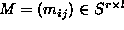(over a totally ordered set S) is sorted, iff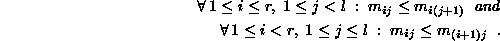Now let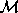be a set of n sorted matrices over S and f be a monotone predicate on S, i.e.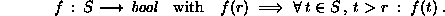If we assume there is any feasible element in one of the matrices in, there certainly is a smallest such element. This is the one we are searching for.

The feasibility test as well as some other parameters can (and have to) be customized through a traits class.

#include <CGAL/sorted_matrix_search.h>

template < class RandomAccessIterator, class Traits >
Traits::Value
 sorted_matrix_search ( RandomAccessIterator f, RandomAccessIterator l, Traits t)

returns the element x in one of the sorted matrices from the range [ f, l ), for which t.is_feasible( x) is true and t.compare( x, y) is true for all other y values from any matrix for which t.is_feasible( y) is true.

Precondition:

1. All matrices in [f, l) are sorted according to Traits::compare_non_strictly.
2. There is at least one entry x in a matrix M[f, l) for which Traits::is_feasible(x) is true.

Requirement:

1. Traits is a model for SortedMatrixSearchTraits.
2. Value type of RandomAccessIterator is Traits::Matrix.

SortedMatrixSearchTraits

### Implementation

The implementation uses an algorithm by Frederickson and Johnson[FJ83, FJ84] and runs in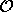(n · k + f · log(n · k)), where n is the number of input matrices, k denotes the maximal dimension of any input matrix and f the time needed for one feasibility test.

### Example

In the following program we build a random vector a = (ai)i = 1,...,5 (elements drawn uniformly from { 0,...,99 }) and construct a Cartesian matrix M containing as elements all sums ai + aj, i,j{1,...,5}. If a is sorted, M is sorted as well. So we can apply sorted_matrix_search to compute the upper bound for the maximal entry of a in M.

```#include <CGAL/Random.h>
#include <CGAL/Cartesian_matrix.h>
#include <CGAL/sorted_matrix_search.h>
#include <CGAL/functional.h>
#include <vector>
#include <algorithm>
#include <iterator>

typedef int                                     Value;
typedef std::vector<Value>                      Vector;
typedef Vector::iterator                        Value_iterator;
typedef std::vector<Vector>                     Vector_cont;
typedef CGAL::Cartesian_matrix<std::plus<int>,
Value_iterator,
Value_iterator>  Matrix;

int main()
{
// set of vectors the matrices are build from:
Vector_cont vectors;

// generate a random vector and sort it:
Vector a;
const int n = 5;
for (int i = 0; i < n; ++i)
a.push_back(CGAL::default_random(100));
std::sort(a.begin(), a.end());
std::cout << "a = ( ";
std::copy(a.begin(), a.end(), std::ostream_iterator<int>(std::cout," "));
std::cout << ")\n";

// build a Cartesian matrix from a:
Matrix M(a.begin(), a.end(), a.begin(), a.end());

// search for an upper bound for max(a):
Value bound = a[n-1];
Value upper_bound =
CGAL::sorted_matrix_search(
&M, &M + 1,advanced